# Improving in-sample and out-of-sample trading system backtesting

## Best Practice Trading System Optimization Series - Part 3 of 4

• Martyn Tinsley
• /
• 12 June, 2018
• /

• Abstract:

• Learn how to squeeze every last drop of value from the walk forward optimization methodology, by maximising (and balancing) the statistical significance across the optimization phases and walk forward phases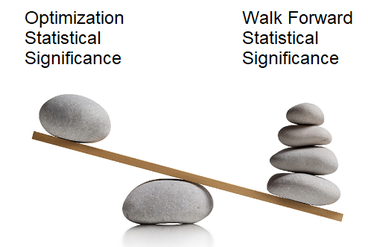This is part 3 of a series of articles covering the subject of best-practice trading system optimization. We highly recommend you read Part 1 and Part 2 first. In part 1 we looked at the importance of statistical significance in all types of back testing, but in particular for Multi-Stage Walk Forward Optimization (MS-WFO). We also started to look at how the problems you will experience as a result of poor statistical significance in your in-sample optimizations, is very different to the problems you will experience as a result of poor statistical significance in your out-of-sample walk-forward tests.

In part 2, we looked at the factors that contribute to statistical significance and therefore how a measure of it might be calculated. We also started to look at real-life practical suggestions of how you can address and improve your statistical significance, leading to the ability to optimize the effectiveness of your trading strategy testing, with the end result being more robust strategies.

In this part we build on this previous thinking to learn how to maximise (and balance) the statistical significance across the optimization phases and walk forward phases of a multi-stage walk forward optimization. The focus here is squeezing every last drop of value from the walk forward optimization methodology.

### Qualitative Definitions

First let’s define some basic qualitative terminology for the purposes of this article (although as it happens, this is also the terminology we have adopted in our walk forward optimization software). We will use a sliding scale of measurement for statistical significance as follows: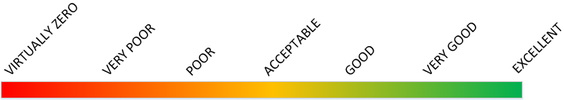Figure 1 - Statistical Significance Definitions

### Unbalanced Walk Forward Optimizations

Above, we alluded to the fact that in order to maximise the effectiveness of your MS-WFO, a process of ‘balancing’ the statistical significance of the optimizations, and the statistical significance of the walk-forward back-tests is required.

If they are not balanced this will result in situations such as those in the following two examples:

### Example 1

OPTIMIZATION STATISTICAL SIGNIFICANCE: EXCELLENT

WALK-FORWARD BACK-TEST STAT SIGNIFICANCE: POOR

In this scenario, the effectiveness of the optimization phases, to identify the best parameters, will be excellent*. However, because the statistical significance of the walk forward validation is classed as ‘poor’, we will not be able to have any confidence in the results obtained in the out-of-sample phases, and will therefore not know whether the chosen parameters will be effective. More precisely we will not know if our system truly has an edge or not.

* Note that just because the effectiveness to select the best parameters is classed as 'excellent' this doesn’t necessarily mean that we are guaranteed to obtain parameters that will make the system profitable. If the trading system does not provide a viable ‘edge’ in the market, excellent parameter selection merely means that you will be able to minimize the losses of the system.

### Example 2

OPTIMIZATION STATISTICAL SIGNIFICANCE: POOR

WALK-FORWARD BACK-TEST STAT SIGNIFICANCE: EXCELLENT

In this scenario, the converse is true. The effectiveness of the model to predict the best parameters is now classed as ‘poor’. If we remember from parts 1 and 2, this means that parameter value selection tends to be based more on randomness and chance, than it does on the effectiveness of the parameters of the trading system. We are therefore very unlikely to obtain the optimal parameters for use in the walk forward validation phases – or in live trading.

However, because the statistical significance of the walk-forward phases is rated as ‘excellent’, this means we can have confidence in the fact that these walk forward results should more closely resemble the results we would achieve if using the selected parameters in live trading (be they good or bad). The issue here however is that the walk-forward back-test results are actually likely to be sub-optimal, because of the fact that the parameters have been selected based on randomness.

### The great ‘balancing act’

It is clear that neither examples 1 or 2 above provide us with an acceptable testing scenario. But what if we could balance the statistical significance by adapting our MS-WFO settings? Remember that one of the major contributing factors that determine the level of statistical significance, is the (uncorrelated) sample size. And providing the system trades fairly consistently over time, the sample size should be approximately proportional to duration. It therefore follows that we should be able to adjust the MS-WFO settings used (primarily the 'number of stages' and the 'optimization to walk forward ratio) in order to produce the balance we need.

Remember that one of the major contributing factors that determine the level of statistical significance, is the sample size (the number of uncorrelated trades).

Key take-away fact

Let’s now assume that by achieving this balance, we could potentially produce the following compromise:

### Example 3

OPTIMIZATION STATISTICAL SIGNIFICANCE: GOOD

WALK-FORWARD BACK-TEST STAT SIGNIFICANCE: GOOD

This presents us with a far more appealing outcome. Here we can be confident that the parameter selection has been undertaken with an ‘good’ level of effectiveness during the optimization phases, meaning that the parameter selection is based more on the performance of the parameters than it is on randomness and chance.

Furthermore we also have an ‘good’ level of trust in the cumulative walk forward validation, meaning we can trade with a level of assurance and confidence that in the long term, actual results should broadly resemble our walk forward expectations from the testing.

### Improving the statistical significance for the MS-WFO

The process of achieving the best possible statistical significance for the MS-WFO is a two-step process as follows:

#### Best Practice MT4 and MT5 EA Optimization Software Walk Forward Pro

Amongst many other features, Walk Forward Pro calculates the statistical significance of both in-sample and out-of-sample phases for you automatically.

Walk Forward Pro helps the user improve the predictive power.of optimizations, leading to more robust systems.

### Step 1 – Achieve better overall statistical significance

You will remember from part 2 that we presented the following two relationships for the statistical significance of the In-Sample (IS) and Out-Of-Sample (OOS) stages: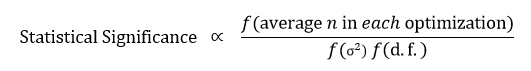Figure 2 - Statistical Significance of the in-sample data set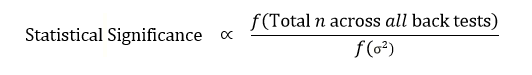Figure 3 - Statistical Significance of the out-of-sample walk forward data set

Based on these two relationships, we can deduce that to increase the overall statistical significance we can do the following:

• #### Reduce the degrees of freedom (number of variables being optimized) which will improve ‘IS’ only

In order to increase the sample size, the obvious solution is to increase the overall duration of the test data being used for the walk forward analysis (since for systems that tend to have a steady rate of trades, the sample size will be roughly proportional to duration). Another tactic to increase sample size is to test the trading system on a shorter timeframe if this is feasible for the system in question (e.g. trading off H1 indicators as opposed to H4). Only you will know if your system is suitable for this approach or not. Do not however, go too low since random noise in the price action will begin to override true movement.

By increasing sample size and reducing the degrees of freedom, while keeping all other WFA settings constant (i.e. keeping the same number of stages, and the same optimization to walk forward ratio), then we will have improved our overall statistical significance, but these will still be unbalanced at this point, hence the need for step 2.

### Step 2 - BalancingThis is the clever part and is in our opinion the ‘art’ of maximising the effectiveness of the MS-WFO methodology.

We perform the balancing by adjusting the number of stages and the ‘optimization to walk forward ratio’. Let’s start with some arbitrary values as follows:

Number of stages = 5
Optimization to Walk Forward Ratio = 3

These settings result in a walk forward analysis that looks like the following:Figure 4 - Walk Forward Analysis - Num Stages = 5, Optimization to Walk Forward Ratio = 3

We can see here that the ‘cumulative’ walk forward validation phase has a longer duration than the individual optimizations (ratio of 5:3). This means the statistical significance of the optimization phases will always be less than that of the WF Validation phases. We have an imbalance. In addition to this, remember that we will usually also have at least one degree of freedom associated to the optimization phase and so based on relationship ① above, this will only worsen the imbalance.

Let’s try to redress this imbalance by adjusting the number of stages and the ‘optimization to walk forward ratio’. We will look at each separately.

### Adjusting the ‘optimization to walk forward ratio’

We used a value of 3 above. Let’s instead use 6 to see what affect this has. We obtain the following: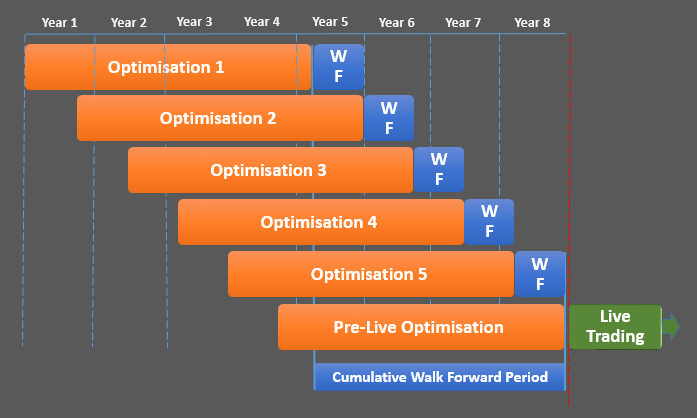Figure 5 - Walk Forward Analysis - Num Stages = 5, Optimization to Walk Forward Ratio = 6

Here, we can clearly see that the ratio of the optimization duration compared with the Cumulative Walk Forward duration has improved. Each optimization epoch is now longer than the cumulative walk forward epoch. In other words, we have started to redress the imbalance. However, given that we will have at least one degree of freedom in the optimization phases, it is almost certain that we will still have an overall imbalance.

### Adjusting the number of Stages

First we return to our initial ‘optimization to walk forward ratio’ of 3. Next we vary the number of stages to see what effect that has on the relative statistical significance. Let’s use say, three stages as an example. The WFO looks as follows: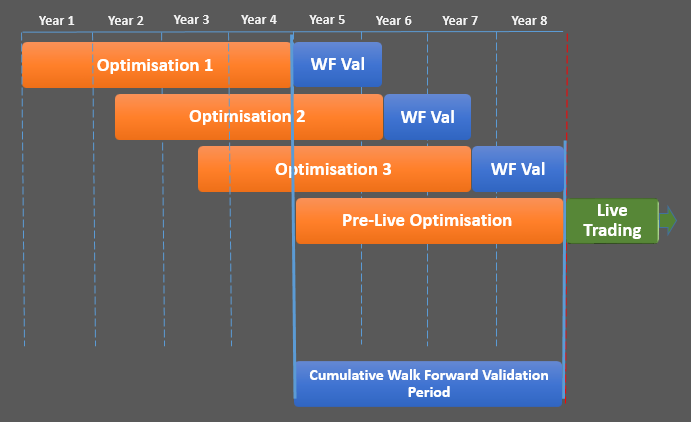Figure 6 - Walk Forward Analysis - Num Stages = 3, Optimization to Walk Forward Ratio = 3

Here we see that the duration of the cumulative walk forward epoch is actually equal to the optimization duration now and so again we have been successful in reducing the imbalance. Again however, given the degrees of freedom issue as mentioned before, we will still have an imbalance.

It is likely that we would have to use a combination of both approaches to achieve an acceptable balance. So in this case, we would need to:

• #### Increase the optimization to walk forward ratio

Important: In the examples above, we have illustrated how the relative durations of the optimizations and walk forward validation phases can be flexed/adjusted. This in turn will affect the relative sample sizes and therefore statistical significance of each. However, remember that in practice, when balancing the statistical significance you also need to account for the number of parameters you are optimizing. The higher the number of parameters, the larger the optimization duration will need to be in comparison to the walk forward validation.

### Conclusions

From the above we can deduce the following.

If Statistical Significance (In-Sample) > Statistical Significance (Out-of-Sample):

• #### Decrease the number of stages

If Statistical Significance (In-Sample) < Statistical Significance (Out-of-Sample):

### Further Considerations

What we have shown above is a very simplistic scenario. Achieving the balance becomes much more complex when optimizing different number of parameters.

Furthermore, there is also a judgement required on the part of the trader as to what the minimum acceptable statistical significance is when compared with the ability to keep a system in sync with changing market conditions (one of the reasons why WFO is so effective). A simple illustration will explain this.

Let’s say you have managed to achieve a balance as follows:

OPTIMIZATION STATISTICAL SIGNIFICANCE: VERY GOOD

WALK-FORWARD BACK-TEST STAT SIGNIFICANCE: VERY GOODFigure 7 - Statistical Significance Definitions

However, what if you had to reduce the number of stages to 1 in order to achieve this? Well if you classify your ‘minimum acceptable statistical significance’ as ‘GOOD’, then it might be preferable to tolerate a slight imbalance in order to use more stages (meaning the WFO would be able to keep your parameters in sync with changing market conditions more effectively). So using 3 stages you might still be able to achieve the following:

OPTIMIZATION STATISTICAL SIGNIFICANCE: GOOD

WALK-FORWARD BACK-TEST STAT SIGNIFICANCE: EXCELLENT

These considerations are complex but if you can master them you will be able to maximise the value of your trading systems. If you are wanting to explore further, you will find the references at the bottom of this page useful.

### In the next article...

In Part 4 we will put the theory we have studied into practice on an actual trading system. This will show a real-life, step-by-step illustration of how results can be significantly improved by taking the approaches we have studied.

## About The Author#### Martyn Tinsley

A passion for all things analytical, and in particular for automated algorithmic trading. Founded Trade Like A Machine to promote best-practice trading system development and optimization techniques, helping other algo traders succeed.

## Spread the word

Like what you've read today? Then please consider sharing

Subscribe to our newsletter

By subscribing you are giving your consent to send you emails in accordance with our privacy policy

We are passionate about algorithmic trading, and about helping other algorithmic traders reach their full potential.

We help traders to develop robust trading systems that deliver results in live accounts.

Popular Posts

June 12, 2018

May 19, 2018